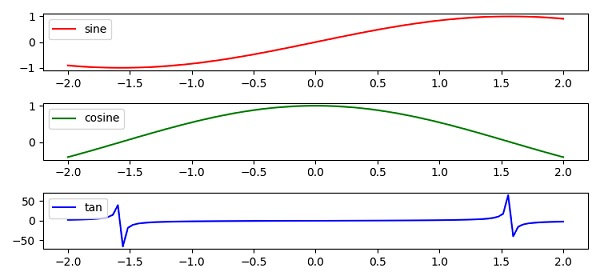# Matplotlib legends in subplot

MatplotlibServer Side ProgrammingProgramming

To add legends in a subplot, we can take the following Steps −

• Using numpy, create points for x, y1, y2 and y3.

• Create a figure and a set of subplots, using the subplots() method, considering 3 subplots.

• Plot the curve on all the subplots(3), with different labels, colors. To place the legend for each curve or subplot adding label.

• To activate label for each curve, use the legend() method.

• To display the figure, use the show() method.

## Example

import numpy as np
from matplotlib import pyplot as plt
plt.rcParams["figure.figsize"] = [7.50, 3.50]
plt.rcParams["figure.autolayout"] = True
x = np.linspace(-2, 2, 100)
y1 = np.sin(x)
y2 = np.cos(x)
y3 = np.tan(x)
f, axes = plt.subplots(3)
axes.plot(x, y1, c='r', label="sine")
axes.legend(loc='upper left')
axes.plot(x, y2, c='g', label="cosine")
axes.legend(loc='upper left')
axes.plot(x, y3, c='b', label="tan")
axes.legend(loc='upper left')
plt.show()

## Output